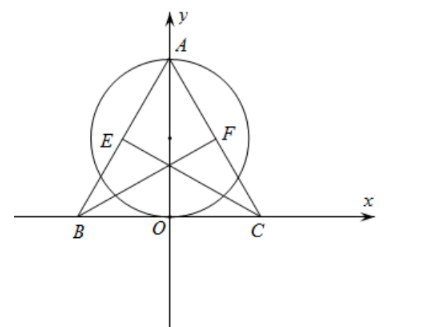【答案】 4

【解析】 解: 以 $B C$ 的中点 $O$ 为坐标原点, $B C$ 所在直线为 $x$ 轴, $O A$ 所在直线为 $y$ 轴, 建立如图所示 的平面直角坐标系.

$4 / 6$